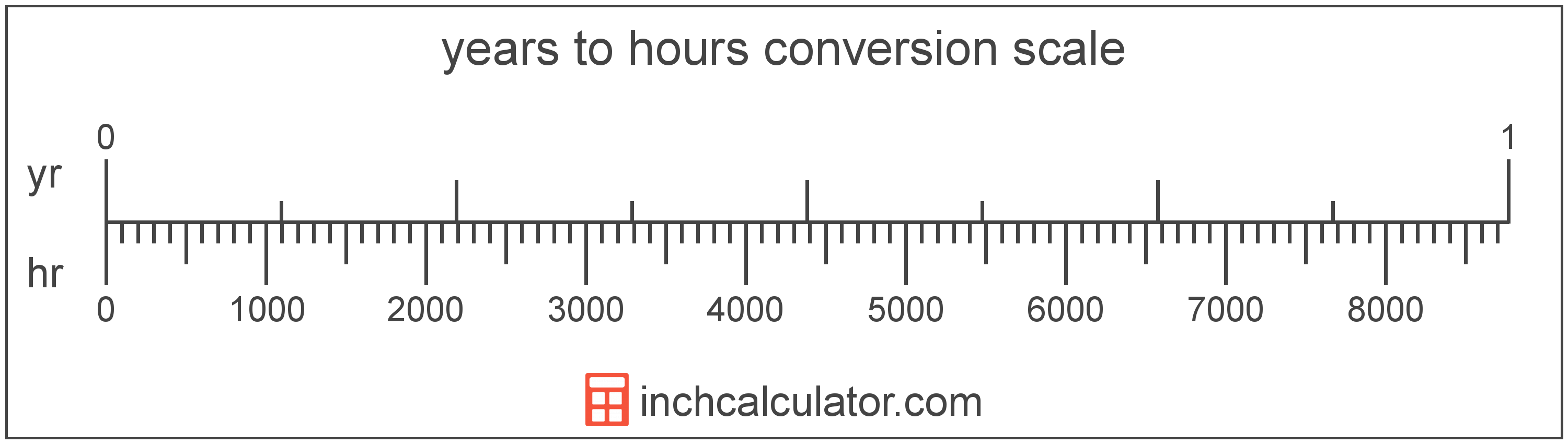# Years to Hours Converter

Enter the time in years below to get the value converted to hours.

Results in Hours:1 yr = 8,765.82 hr

Do you want to convert hours to years?

## How to Convert Years to Hours

To convert a measurement in years to a measurement in hours, multiply the time by the following conversion ratio: 8,765.82 hours/year.

Since one year is equal to 8,765.82 hours, you can use this simple formula to convert:

hours = years × 8,765.82

The time in hours is equal to the time in years multiplied by 8,765.82.

For example, here's how to convert 5 years to hours using the formula above.
hours = (5 yr × 8,765.82) = 43,829.1 hr### How Many Hours Are in a Year?

There are 8,765.82 hours in a year, which is why we use this value in the formula above.

1 yr = 8,765.82 hr

## What Is a Year?

One year is the time it takes for the Earth to complete its orbit around the Sun. There are 365 days in a year, with the exception of the leap year when there are 366.

We have leap years because the Earth actually takes 365.2425 days to revolve around the sun, but we round to 365 for convenience. However, since we miss a quarter of a day per revolution around the sun, this means that we add an extra day to the year once every 4 years, hence the leap year.

Years can be abbreviated as yr (plural yrs), and are also sometimes abbreviated as y. For example, 1 year can be written as 1 yr or 1 y, and 2 years can be written as 2 yrs.

## What Is an Hour?

The hour is a period of time equal to 1/24 of a day or 60 minutes.

The hour is an SI accepted unit for time for use with the metric system. Hours can be abbreviated as hr (plural hrs); for example, 1 hour can be written as 1 hr, and 2 hours can be written as 2 hrs.

## Year to Hour Conversion Table

Table showing various year measurements converted to hours.
Years Hours
0.001 yr 8.7658 hr
0.002 yr 17.53 hr
0.003 yr 26.3 hr
0.004 yr 35.06 hr
0.005 yr 43.83 hr
0.006 yr 52.59 hr
0.007 yr 61.36 hr
0.008 yr 70.13 hr
0.009 yr 78.89 hr
0.01 yr 87.66 hr
0.02 yr 175.32 hr
0.03 yr 262.97 hr
0.04 yr 350.63 hr
0.05 yr 438.29 hr
0.06 yr 525.95 hr
0.07 yr 613.61 hr
0.08 yr 701.27 hr
0.09 yr 788.92 hr
0.1 yr 876.58 hr
0.2 yr 1,753 hr
0.3 yr 2,630 hr
0.4 yr 3,506 hr
0.5 yr 4,383 hr
0.6 yr 5,259 hr
0.7 yr 6,136 hr
0.8 yr 7,013 hr
0.9 yr 7,889 hr
1 yr 8,766 hr